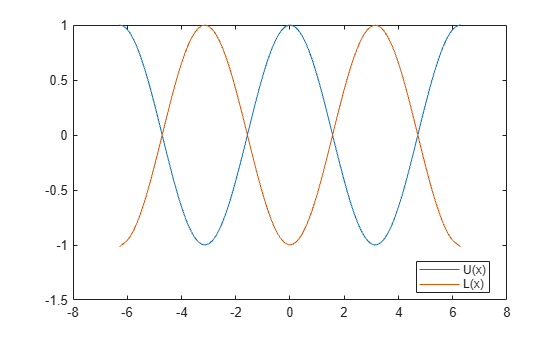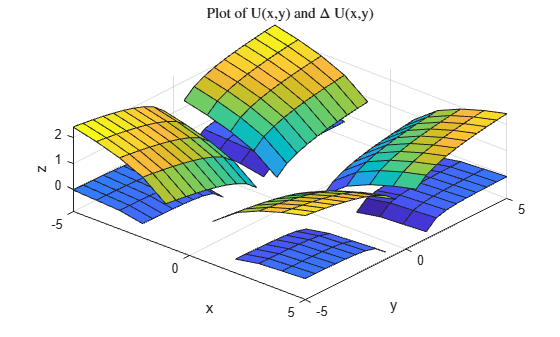# del2

Discrete Laplacian

## Syntax

``L = del2(U)``
``L = del2(U,h)``
``L = del2(U,hx,hy,...,hN)``

## Description

example

````L = del2(U)` returns a discrete approximation of Laplace’s differential operator applied to `U` using the default spacing, `h = 1`, between all points.```

example

````L = del2(U,h)` specifies a uniform, scalar spacing, `h`, between points in all dimensions of `U`.```

example

````L = del2(U,hx,hy,...,hN)` specifies the spacing `hx,hy,...,hN` between points in each dimension of `U`. Specify each spacing input as a scalar or a vector of coordinates. The number of spacing inputs must equal the number of dimensions in `U`. The first spacing value `hx` specifies the x-spacing (as a scalar) or x-coordinates (as a vector) of the points. If it is a vector, its length must be equal to `size(U,2)`.The second spacing value `hy` specifies the y-spacing (as a scalar) or y-coordinates (as a vector) of the points. If it is a vector, its length must be equal to `size(U,1)`.All other spacing values specify the spacing (as scalars) or coordinates (as vectors) of the points in the corresponding dimension in `U`. If, for `n > 2`, the `n`th spacing input is a vector, then its length must be equal to `size(U,n)`. ```

## Examples

collapse all

Calculate the acceleration of an object from a vector of position data.

Create a vector of position data.

`p = [1 3 6 10 16 18 29];`

To find the acceleration of the object, use `del2` to calculate the second numerical derivative of `p`. Use the default spacing `h = 1` between data points.

`L = 4*del2(p)`
```L = 1×7 1 1 1 2 -4 9 22 ```

Each value of `L` is an approximation of the instantaneous acceleration at that point.

Calculate the discrete 1-D Laplacian of a cosine vector.

Define the domain of the function.

`x = linspace(-2*pi,2*pi);`

This produces 100 evenly spaced points in the range $-2\pi \le x\le 2\pi$.

Create a vector of cosine values in this domain.

`U = cos(x);`

Calculate the Laplacian of `U` using `del2`. Use the domain vector `x` to define the 1-D coordinate of each point in `U`.

`L = 4*del2(U,x);`

Analytically, the Laplacian of this function is equal to $\Delta U=-\mathrm{cos}\left(x\right)$.

Plot the results.

```plot(x,U,x,L) legend('U(x)','L(x)','Location','Best')```The graph of `U` and `L` agrees with the analytic result for the Laplacian.

Calculate and plot the discrete Laplacian of a multivariate function.

Define the x and y domain of the function.

`[x,y] = meshgrid(-5:0.25:5,-5:0.25:5);`

Define the function $U\left(x,y\right)=\frac{1}{3}\left({x}^{4}+{y}^{4}\right)$ over this domain.

`U = 1/3.*(x.^4+y.^4);`

Calculate the Laplacian of this function using `del2`. The spacing between the points in `U` is equal in all directions, so you can specify a single spacing input, `h`.

```h = 0.25; L = 4*del2(U,h);```

Analytically, the Laplacian of this function is equal to $\Delta U\left(x,y\right)=4{x}^{2}+4{y}^{2}$.

Plot the discrete Laplacian, `L`.

```figure surf(x,y,L) grid on title('Plot of \$\Delta U(x,y) = 4x^2+4y^2\$','Interpreter','latex') xlabel('x') ylabel('y') zlabel('z') view(35,14)```The graph of `L` agrees with the analytic result for the Laplacian.

Calculate the discrete Laplacian of a natural logarithm function.

Define the x and y domain of the function on a grid of real numbers.

`[x,y] = meshgrid(-5:5,-5:0.5:5);`

Define the function $U\left(x,y\right)=\frac{1}{2}\mathrm{log}\left({x}^{2}y\right)$ over this domain.

`U = 0.5*log(x.^2.*y);`

The logarithm is complex-valued when the argument `y` is negative.

Use `del2` to calculate the discrete Laplacian of this function. Specify the spacing between grid points in each direction.

```hx = 1; hy = 0.5; L = 4*del2(U,hx,hy);```

Analytically, the Laplacian is equal to $\Delta U\left(x,y\right)=-\left(1/{x}^{2}+1/2{y}^{2}\right)$. This function is not defined on the lines $x=0$ or $y=0$.

Plot the real parts of `U` and `L` on the same graph.

```figure surf(x,y,real(L)) hold on surf(x,y,real(U)) grid on title('Plot of U(x,y) and \$\Delta\$ U(x,y)','Interpreter','latex') xlabel('x') ylabel('y') zlabel('z') view(41,58)```The top surface is `U` and the bottom surface is `L`.

## Input Arguments

collapse all

Input array, specified as a vector, matrix, or multidimensional array.

Data Types: `single` | `double`
Complex Number Support: Yes

Spacing in all dimensions, specified as `1` (default), or a scalar.

Data Types: `single` | `double`
Complex Number Support: Yes

Spacing in each dimension, specified as separate arguments of scalars (for uniform spacing) or vectors (for nonuniform spacing). The number of spacing inputs must be equal to the number of dimensions in `U`. Each spacing input defines the spacing between points in one dimension of `U`:

• The first spacing value `hx` specifies the x-spacing (as a scalar) or x-coordinates (as a vector) of the points. If it is a vector, its length must be equal to `size(U,2)`.

• The second spacing value `hy` specifies the y-spacing (as a scalar) or y-coordinates (as a vector) of the points. If it is a vector, its length must be equal to `size(U,1)`.

• All other spacing values specify the spacing (as scalars) or coordinates (as vectors) of the points in the corresponding dimension in `U`. If, for ```n > 2```, the `n`th spacing input is a vector, then its length must be equal to `size(U,n)`.

Data Types: `single` | `double`
Complex Number Support: Yes

## Output Arguments

collapse all

Discrete Laplacian approximation, returned as a vector, matrix, or multidimensional array. `L` is the same size as the input, `U`.

collapse all

### Laplace’s differential operator

The definition of the Laplace operator used by `del2` in MATLAB® depends on the dimensionality of the data in `U`.

• If `U` is a vector representing a function U(x) that is evaluated on the points of a line, then `del2(U)` is a finite difference approximation of

`$L=\frac{\Delta U}{4}=\frac{1}{4}\frac{{\partial }^{2}U}{\partial {x}^{2}}.$`
• If `U` is a matrix representing a function U(x,y) that is evaluated at the points of a square grid, then `del2(U)` is a finite difference approximation of

`$L=\frac{\Delta U}{4}=\frac{1}{4}\left(\frac{{\partial }^{2}U}{\partial {x}^{2}}+\frac{{\partial }^{2}U}{\partial {y}^{2}}\right).$`
• For functions of three or more variables, U(x,y,z,...), the discrete Laplacian `del2(U)` calculates second-derivatives in each dimension,

`$L=\frac{\Delta U}{2N}=\frac{1}{2N}\left(\frac{{\partial }^{2}U}{\partial {x}^{2}}+\frac{{\partial }^{2}U}{\partial {y}^{2}}+\frac{{\partial }^{2}U}{\partial {z}^{2}}+...\right),$`

where N is the number of dimensions in U and $N\ge 2$.

## Algorithms

If the input `U` is a matrix, the interior points of `L` are found by taking the difference between a point in `U` and the average of its four neighbors:

`${L}_{ij}=\left[\frac{\left({u}_{i+1,j}+{u}_{i-1,j}+{u}_{i,j+1}+{u}_{i,j-1}\right)}{4}-{u}_{i,j}\right]\text{\hspace{0.17em}}.$`

Then, `del2` calculates the values on the edges of `L` by linearly extrapolating the second differences from the interior. This formula is extended for multidimensional `U`.